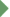Chapter 3 ContentsBeginning How Does Achievement Differ Across Mathematics Content Areas? In Which Content Areas Are Students Relatively Strong or Weak? What Are the Gender Differences in Achievement for the Content Areas?

© 2001 International Association for the Evaluation of Educational Achievement (IEA)

####Chapter 3 presents results by the major content areas in mathematics to provide information about the possible effects of curricular variation on average achievement. Average performance is provided for five content areas: fractions and number sense; measurement; data representation, analysis, and probability; geometry; and algebra.

As delineated by the curriculum of the countries around the world and in the Benchmarking entities, mathematics contains a range of content areas (see Chapter 5 on curriculum). For example, almost all TIMSS 1999 countries and Benchmarking participants reported some elements of arithmetic as well as algebra and geometry in the eighth-grade mathematics curriculum. Since these content areas can differ in complexity, enter the curriculum at different times, receive varying degrees of emphasis, or even be taught as separate courses, Chapter 3 presents results by the major content areas in mathematics. For each Benchmarking entity, average achievement is shown for each content area and compared with the international average for that content area, and average achievement in the content areas is profiled in relation to overall mathematics achievement. Results are also provided by gender. These different perspectives are provided to identify the relative strengths and weaknesses of students in the different mathematics content areas as well as the possible effects of curricular variation on average achievement.

The TIMSS 1999 mathematics test for the eighth grade was designed to enable reporting by five content areas in accordance with the TIMSS mathematics framework. These areas, with their main topics, are:

 • Fractions and number sense Includes whole numbers, fractions and decimals, integers, exponents, estimation and approximation, proportionality • Measurement Includes standard and non-standard units, common measures, perimeter, area, volume, estimation of measures • Data representation, analysis, and probability Includes representing and interpreting tables, charts, and graphs; range, mean; informal likelihood, simple numerical probability • Geometry Includes points, lines, planes, angles, visualization, triangles, polygons, circles, transformations, symmetry, congruence, similarity, constructions • Algebra Includes number patterns, representation of numerical situations, solving simple linear equations, operations with expressions, representations of relations and functions.TIMSS 1999 is a project of the International Study Center
Boston College, Lynch School of Education